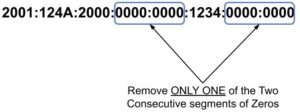## 1. Introduction

When we design a network, we always need to know how many addresses do we need in this network. Also, we would like to know the range of IP addresses in our network so that we can assign an address for each device in the network.

In this tutorial, we’re going to show a simple method to find the range of IP addresses given a subnet mask.

## 2. Problem Explanation

In IPv4, the IP address consists of 32 bits number. And we represent it by 4 octets (8-bits each). Usually, when we refer to a network, we also refer to a subnet. This subnet can be a number (for example /24), and we can write it like an IP address (example /255.255.255.0). From this subnet, we’d like to find out what are the IP addresses in this network.

For example, if we have a network 192.168.0.1/24 (or with subnet 255.255.255.0), then we have 256 usable addresses from 192.168.0.0 up to 192.168.0.255.

## 3. Algorithm Idea

In general, if we deal with the IP address as a 32-bit number, and we have a subnet mask of, then the subnet mask theoretically can take values between. Then, we have two things to estimate, what are the number of possible addresses with the given subnet mask, and what is the starting address.

We can get the number of possible addresses easily by the following formula. That means if we have a subnet mask of, then the possible addresses are. And if we have a subnet mask of, then the possible number of addresses is, which means the given address is the only possible address in this case. One more example the case of subnet mask of, we will haveaddresses.

Then, we need to interpret this subnet mask in the octets form. If we have a subnetmask of, we are actually having a 32-bit number that has the left most 24-bits as ones and the rest are zeros:From the previous table, we can see how the subnet mask can be interpreted. So, if we have an IP address like 192.168.0.10/24, we can write it down in a table to show the binary octets compared to:To find the starting address in the following subnet mask, we simply do binary “and” operation between the IP address and the subnet mask:Finally, we calculate the last IP address by applying the “or” operation on it with the bitwise binary inverse of the subnet mask to the first IP address:With these simple steps, we know how to find the number of possible IP addresses. We can also find the first and last IP addresses and the range becomes from 192.168.0.0 up to 192.168.0.255.

## 6. Examples

The same idea happens if we have a subnet mask that is not exactly at one of the octets. Let’s see and example of 192.168.0.10/30. By following the same idea we havepossible IP addresses:Let’s take one more example where the subnet mask is not in the last octet like 10.0.0.0/20. In this case we havepossible IP address:Luckily, in most programming languages, we don’t need to convert between binary and decimal to do the binary operations. So, we can apply the binary operations directly on most countable numbers (like integers or chars). In other words, we can represent the IP address by a 32-bit number or usingdigits 8-bits each (like characters or bytes).

## 7. IPv6

In IPv6, the same idea for the subnet is similar to the IPv4. But the difference is in the size of the address and the allowed range. The IPv6 address hasbits compared tobits in IPv4. The address is represented insegments compared tooctets in the IPv4. Each segment is 4 hex numbers taking range fromtocompared to 8-bits per octet in IPv4 with the decimal rangeto. The separation between blocks in IPv6 is a colon (:) compared to dot (.) used in IPv4.

In general, we can get the number of the available IP addresses the same way we did it in IPv4. So, if we have a subnet of size, then we haveavailable IP addresses. And we can get the first one of them by applying bitwise and with a mask of sizebits, where the firstbits are ones and the rest are zeros. And we can get the last address by adding the first address to the inverse of the mask (bits with the firstbits as zeros and the rest are ones).

Let’s see an IPv6 example for. Notice that each segment is written as 4 hex digits which equal 16-binary bits. The number of addresses will be:Notice thatis equivalent toones. Another note is that the IPv6 address can also take short forms by removing leading zeros in any segment.

Thus, we write our example first address:And another shortened form is to replace one of the sequences of empty segments (all zero segments) with a double colon. So, the other shortened form of this address becomes:Note that the shortened version does not allow more than one time for omitting the zeros segments. So, if we have and address:The shortened version will be:Or:In this shortened version, we can know how many zeros are removed because we know that we have 128 bits divided into 8 segments. Thus, we know how many segments are removed from the 8 and these are the zeros omitted.

## 8. Complexity

The time and space complexities of finding the range of IP addresses arebecause we just need to put the subnet mask in a formula to find the range.